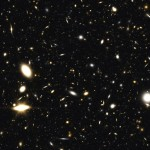# Theory of Selfvariations (TSV) (Emmanuil Manousos)The Theory of Selfvariations (TSV) is a physical theory originated by the Greek mathematician Emmanuil Manousos in 2007. It relies on the assumption of a continuous slight increase of the rest mass and electric charge of material particles called the selfvariations. The selfvariations are strictly defined by the law of the selfvariations and are consistent with the fundamental principles of physics.

Save

### Cosmology

The law of Selfvariations justifies the hitherto known cosmological data. For the beginning of the universe it predicts a state slightly different from the vacuum. Because of the Selfvariations the universe shifted from the initial vacuum state to the form we observe today. The cosmological model of the selfvariations is a self-consistent static cosmological model. Furthermore, an implication of the law is that gravity cannot play the role attributed to it by the SCM. At cosmological scales we come to the conclusion that gravity cannot lead the universe neither to collapse nor to expansion.

### Interaction of material particles

The TSV predicts one interaction with a common mechanism between material particles (Unified Selfvariation Interaction, USVI). The USVI is the sum of three terms, of which everyone plays a different role in the interaction. The first is expressed by a force parallel to the direction of movement of the material particle and is exclusively the result of the Selfvariations. The second term expresses the curvature of space-time and the third the Lorentz force. The characterizing properties of the interaction depend on the relative strength of the three terms. The distinction between individual interactions is due to the different argument i.e. the selfvariating ‘load’ which can be the rest mass, the electric charge, etc. which causes the interaction in the first place.

### The wave equation of the TSV

The TSV provides a wave equation whose special cases are the equations of Maxwell, the Schrodinger equation and relevant wave equations. It clearly demonstrates the particle structure and wave behavior of matter and the relationship between them.

### On the conserved physical quantities

One of the most important conclusions of the TSV is that the energy, momentum and angular momentum of the material particle are distributed in spacetime. The study of this distribution shows an overall mathematical expression for the conserved physical quantities. Furthermore TSV gives the four-vector of current density of the conserved physical quantities. Note that one of the conserved physical quantities is dimensionless, i.e. a complex number.

### On the particle structure of matter

Among the equations of the TSV we find sums of squares equal to zero. One of these sums gives important information on the particle structure of matter. This equation relates fundamental constants of the TSV. The combination of this equation with the mathematical expression of the four-vector of the conserved physical quantities gives a method for calculating the rest mass of material particles.The JWST may test the validity of the TSV.

Photo: Simulated view of the deep universe as seen through the James Webb Space Telescope (JWST, planned launch date in 2018).

### Books

• Manousos, E. (2011). “The Theory of Selfvariations”, Hellenic Physical Society Publications, Athens 2011, ISBN 978-960-9457-06-4

#### Photo Credits

1. NASA/ESA, Andrew Benson (University of Durham, United Kingdom), and the JWST Science Team (STScI)